College Algebra 2e

# 4.1Linear Functions

College Algebra 2e4.1 Linear Functions

### Learning Objectives

In this section, you will:

• Represent a linear function.
• Determine whether a linear function is increasing, decreasing, or constant.
• Interpret slope as a rate of change.
• Write and interpret an equation for a linear function.
• Graph linear functions.
• Determine whether lines are parallel or perpendicular.
• Write the equation of a line parallel or perpendicular to a given line.
Figure 1 Shanghai MagLev Train (credit: "kanegen"/Flickr)

Just as with the growth of a bamboo plant, there are many situations that involve constant change over time. Consider, for example, the first commercial maglev train in the world, the Shanghai MagLev Train (Figure 1). It carries passengers comfortably for a 30-kilometer trip from the airport to the subway station in only eight minutes2.

Suppose a maglev train travels a long distance, and maintains a constant speed of 83 meters per second for a period of time once it is 250 meters from the station. How can we analyze the train’s distance from the station as a function of time? In this section, we will investigate a kind of function that is useful for this purpose, and use it to investigate real-world situations such as the train’s distance from the station at a given point in time.

### Representing Linear Functions

The function describing the train’s motion is a linear function, which is defined as a function with a constant rate of change. This is a polynomial of degree 1. There are several ways to represent a linear function, including word form, function notation, tabular form, and graphical form. We will describe the train’s motion as a function using each method.

#### Representing a Linear Function in Word Form

Let’s begin by describing the linear function in words. For the train problem we just considered, the following word sentence may be used to describe the function relationship.

• The train’s distance from the station is a function of the time during which the train moves at a constant speed plus its original distance from the station when it began moving at constant speed.

The speed is the rate of change. Recall that a rate of change is a measure of how quickly the dependent variable changes with respect to the independent variable. The rate of change for this example is constant, which means that it is the same for each input value. As the time (input) increases by 1 second, the corresponding distance (output) increases by 83 meters. The train began moving at this constant speed at a distance of 250 meters from the station.

#### Representing a Linear Function in Function Notation

Another approach to representing linear functions is by using function notation. One example of function notation is an equation written in the slope-intercept form of a line, where $x x$ is the input value, $m m$ is the rate of change, and $b b$ is the initial value of the dependent variable.

In the example of the train, we might use the notation $D(t) D(t)$ where the total distance $D D$ is a function of the time $t. t.$ The rate, $m, m,$ is 83 meters per second. The initial value of the dependent variable $b b$ is the original distance from the station, 250 meters. We can write a generalized equation to represent the motion of the train.

$D(t)=83t+250 D(t)=83t+250$

#### Representing a Linear Function in Tabular Form

A third method of representing a linear function is through the use of a table. The relationship between the distance from the station and the time is represented in Figure 2. From the table, we can see that the distance changes by 83 meters for every 1 second increase in time.

Figure 2 Tabular representation of the function $D D$ showing selected input and output values

### Q&A

Can the input in the previous example be any real number?

No. The input represents time so while nonnegative rational and irrational numbers are possible, negative real numbers are not possible for this example. The input consists of non-negative real numbers.

#### Representing a Linear Function in Graphical Form

Another way to represent linear functions is visually, using a graph. We can use the function relationship from above, $D(t)=83t+250, D(t)=83t+250,$ to draw a graph as represented in Figure 3. Notice the graph is a line. When we plot a linear function, the graph is always a line.

The rate of change, which is constant, determines the slant, or slope of the line. The point at which the input value is zero is the vertical intercept, or y-intercept, of the line. We can see from the graph that the y-intercept in the train example we just saw is $(0,250) (0,250)$ and represents the distance of the train from the station when it began moving at a constant speed.

Figure 3 The graph of $D(t)=83t+250 D(t)=83t+250$ . Graphs of linear functions are lines because the rate of change is constant.

Notice that the graph of the train example is restricted, but this is not always the case. Consider the graph of the line $f(x)=2x+1. f(x)=2x+1.$ Ask yourself what numbers can be input to the function. In other words, what is the domain of the function? The domain is comprised of all real numbers because any number may be doubled, and then have one added to the product.

### Linear Function

A linear function is a function whose graph is a line. Linear functions can be written in the slope-intercept form of a line

$f(x)=mx+b f(x)=mx+b$

where $b b$ is the initial or starting value of the function (when input, $x=0 x=0$ ), and $m m$ is the constant rate of change, or slope of the function. The y-intercept is at $(0,b). (0,b).$

### Example 1

#### Using a Linear Function to Find the Pressure on a Diver

The pressure, $P, P,$ in pounds per square inch (PSI) on the diver in Figure 4 depends upon her depth below the water surface, $d, d,$ in feet. This relationship may be modeled by the equation, $P(d)=0.434d+14.696. P(d)=0.434d+14.696.$ Restate this function in words.

Figure 4 (credit: Ilse Reijs and Jan-Noud Hutten)

#### Analysis

The initial value, 14.696, is the pressure in PSI on the diver at a depth of 0 feet, which is the surface of the water. The rate of change, or slope, is 0.434 PSI per foot. This tells us that the pressure on the diver increases 0.434 PSI for each foot her depth increases.

### Determining Whether a Linear Function Is Increasing, Decreasing, or Constant

The linear functions we used in the two previous examples increased over time, but not every linear function does. A linear function may be increasing, decreasing, or constant. For an increasing function, as with the train example, the output values increase as the input values increase. The graph of an increasing function has a positive slope. A line with a positive slope slants upward from left to right as in Figure 5(a). For a decreasing function, the slope is negative. The output values decrease as the input values increase. A line with a negative slope slants downward from left to right as in Figure 5(b). If the function is constant, the output values are the same for all input values so the slope is zero. A line with a slope of zero is horizontal as in Figure 5(c).

Figure 5

### Increasing and Decreasing Functions

The slope determines if the function is an increasing linear function, a decreasing linear function, or a constant function.

• $f(x)=mx+b f(x)=mx+b$ is an increasing function if $m>0. m>0.$
• $f(x)=mx+b f(x)=mx+b$ is a decreasing function if $m<0. m<0.$
• $f(x)=mx+b f(x)=mx+b$ is a constant function if $m=0. m=0.$

### Example 2

#### Deciding Whether a Function Is Increasing, Decreasing, or Constant

Studies from the early 2010s indicated that teens sent about 60 texts a day, while more recent data indicates much higher messaging rates among all users, particularly considering the various apps with which people can communicate.3. For each of the following scenarios, find the linear function that describes the relationship between the input value and the output value. Then, determine whether the graph of the function is increasing, decreasing, or constant.

1. The total number of texts a teen sends is considered a function of time in days. The input is the number of days, and output is the total number of texts sent.
2. A person has a limit of 500 texts per month in their data plan. The input is the number of days, and output is the total number of texts remaining for the month.

### Example 7

#### Writing an Equation for a Linear Function Given Two Points

If $f f$ is a linear function, with $f(3)=−2, f(3)=−2,$ and $f(8)=1, f(8)=1,$ find an equation for the function in slope-intercept form.

### Try It #3

If $f(x) f(x)$ is a linear function, with $f(2)=–11, f(2)=–11,$ and $f(4)=−25, f(4)=−25,$ write an equation for the function in slope-intercept form.

### Modeling Real-World Problems with Linear Functions

In the real world, problems are not always explicitly stated in terms of a function or represented with a graph. Fortunately, we can analyze the problem by first representing it as a linear function and then interpreting the components of the function. As long as we know, or can figure out, the initial value and the rate of change of a linear function, we can solve many different kinds of real-world problems.

### How To

Given a linear function $f f$ and the initial value and rate of change, evaluate $f( c ). f( c ).$

1. Determine the initial value and the rate of change (slope).
2. Substitute the values into $f(x)=mx+b. f(x)=mx+b.$
3. Evaluate the function at $x=c. x=c.$

### Example 8

#### Using a Linear Function to Determine the Number of Songs in a Music Collection

Marcus currently has 200 songs in his music collection. Every month, he adds 15 new songs. Write a formula for the number of songs, $N, N,$ in his collection as a function of time, $t, t,$ the number of months. How many songs will he own at the end of one year?

#### Analysis

Notice that N is an increasing linear function. As the input (the number of months) increases, the output (number of songs) increases as well.

### Example 9

#### Using a Linear Function to Calculate Salary Based on Commission

Working as an insurance salesperson, Ilya earns a base salary plus a commission on each new policy. Therefore, Ilya’s weekly income $I, I,$ depends on the number of new policies, $n, n,$ he sells during the week. Last week he sold 3 new policies, and earned $760 for the week. The week before, he sold 5 new policies and earned$920. Find an equation for $I(n), I(n),$ and interpret the meaning of the components of the equation.

### Example 10

#### Using Tabular Form to Write an Equation for a Linear Function

Table 1 relates the number of rats in a population to time, in weeks. Use the table to write a linear equation.

 number of weeks, w 0 2 4 6 number of rats, P(w) 1000 1080 1160 1240
Table 1

### Q&A

Is the initial value always provided in a table of values like Table 1?

No. Sometimes the initial value is provided in a table of values, but sometimes it is not. If you see an input of 0, then the initial value would be the corresponding output. If the initial value is not provided because there is no value of input on the table equal to 0, find the slope, substitute one coordinate pair and the slope into $f(x)=mx+b, f(x)=mx+b,$ and solve for $b. b.$

### Try It #4

A new plant food was introduced to a young tree to test its effect on the height of the tree. Table 2 shows the height of the tree, in feet, $x x$ months since the measurements began. Write a linear function, $H(x), H(x),$ where $x x$ is the number of months since the start of the experiment.

 x 0 2 4 8 12 H(x) 12.5 13.5 14.5 16.5 18.5
Table 2

### Graphing Linear Functions

Now that we’ve seen and interpreted graphs of linear functions, let’s take a look at how to create the graphs. There are three basic methods of graphing linear functions. The first is by plotting points and then drawing a line through the points. The second is by using the y-intercept and slope. And the third method is by using transformations of the identity function $f(x)=x. f(x)=x.$

#### Graphing a Function by Plotting Points

To find points of a function, we can choose input values, evaluate the function at these input values, and calculate output values. The input values and corresponding output values form coordinate pairs. We then plot the coordinate pairs on a grid. In general, we should evaluate the function at a minimum of two inputs in order to find at least two points on the graph. For example, given the function, $f(x)=2x, f(x)=2x,$ we might use the input values 1 and 2. Evaluating the function for an input value of 1 yields an output value of 2, which is represented by the point $(1,2). (1,2).$ Evaluating the function for an input value of 2 yields an output value of 4, which is represented by the point $(2,4). (2,4).$ Choosing three points is often advisable because if all three points do not fall on the same line, we know we made an error.

### How To

Given a linear function, graph by plotting points.

1. Choose a minimum of two input values.
2. Evaluate the function at each input value.
3. Use the resulting output values to identify coordinate pairs.
4. Plot the coordinate pairs on a grid.
5. Draw a line through the points.

### Example 11

#### Graphing by Plotting Points

Graph $f(x)=− 2 3 x+5 f(x)=− 2 3 x+5$ by plotting points.

#### Analysis

The graph of the function is a line as expected for a linear function. In addition, the graph has a downward slant, which indicates a negative slope. This is also expected from the negative, constant rate of change in the equation for the function.

### Try It #5

Graph $f(x)=− 3 4 x+6 f(x)=− 3 4 x+6$ by plotting points.

#### Graphing a Function Using y-intercept and Slope

Another way to graph linear functions is by using specific characteristics of the function rather than plotting points. The first characteristic is its y-intercept, which is the point at which the input value is zero. To find the y-intercept, we can set $x=0 x=0$ in the equation.

The other characteristic of the linear function is its slope.

Let’s consider the following function.

$f(x)= 1 2 x+1 f(x)= 1 2 x+1$

The slope is $1 2 . 1 2 .$ Because the slope is positive, we know the graph will slant upward from left to right. The y-intercept is the point on the graph when $x=0. x=0.$ The graph crosses the y-axis at $(0,1). (0,1).$ Now we know the slope and the y-intercept. We can begin graphing by plotting the point $(0,1). (0,1).$ We know that the slope is the change in the y-coordinate over the change in the x-coordinate. This is commonly referred to as rise over run, $m= rise run . m= rise run .$ From our example, we have $m= 1 2 , m= 1 2 ,$ which means that the rise is 1 and the run is 2. So starting from our y-intercept $(0,1), (0,1),$ we can rise 1 and then run 2, or run 2 and then rise 1. We repeat until we have a few points, and then we draw a line through the points as shown in Figure 12.

Figure 12

### Graphical Interpretation of a Linear Function

In the equation $f(x)=mx+b f(x)=mx+b$

• $b b$ is the y-intercept of the graph and indicates the point $(0,b) (0,b)$ at which the graph crosses the y-axis.
• $m m$ is the slope of the line and indicates the vertical displacement (rise) and horizontal displacement (run) between each successive pair of points. Recall the formula for the slope:

### Q&A

Do all linear functions have y-intercepts?

Yes. All linear functions cross the y-axis and therefore have y-intercepts. (Note: A vertical line is parallel to the y-axis does not have a y-intercept, but it is not a function.)

### How To

Given the equation for a linear function, graph the function using the y-intercept and slope.

1. Evaluate the function at an input value of zero to find the y-intercept.
2. Identify the slope as the rate of change of the input value.
3. Plot the point represented by the y-intercept.
4. Use $rise run rise run$ to determine at least two more points on the line.
5. Sketch the line that passes through the points.

### Example 12

#### Graphing by Using the y-intercept and Slope

Graph $f(x)=− 2 3 x+5 f(x)=− 2 3 x+5$ using the y-intercept and slope.

#### Analysis

The graph slants downward from left to right, which means it has a negative slope as expected.

### Try It #6

Find a point on the graph we drew in Example 12 that has a negative x-value.

#### Graphing a Function Using Transformations

Another option for graphing is to use a transformation of the identity function $f(x)=x. f(x)=x.$ A function may be transformed by a shift up, down, left, or right. A function may also be transformed using a reflection, stretch, or compression.

##### Vertical Stretch or Compression

In the equation $f(x)=mx, f(x)=mx,$ the $m m$ is acting as the vertical stretch or compression of the identity function. When $m m$ is negative, there is also a vertical reflection of the graph. Notice in Figure 14 that multiplying the equation of $f(x)=x f(x)=x$ by $m m$ stretches the graph of $f f$ by a factor of $m m$ units if $m>1 m>1$ and compresses the graph of $f f$ by a factor of $m m$ units if $0 This means the larger the absolute value of $m, m,$ the steeper the slope.

Figure 14 Vertical stretches and compressions and reflections on the function $f(x)=x f(x)=x$
##### Vertical Shift

In $f(x)=mx+b, f(x)=mx+b,$ the $b b$ acts as the vertical shift, moving the graph up and down without affecting the slope of the line. Notice in Figure 15 that adding a value of $b b$ to the equation of $f( x )=x f( x )=x$ shifts the graph of $f f$ a total of $b b$ units up if $b b$ is positive and $|b| |b|$ units down if $b b$ is negative.

Figure 15 This graph illustrates vertical shifts of the function $f(x)=x. f(x)=x.$

Using vertical stretches or compressions along with vertical shifts is another way to look at identifying different types of linear functions. Although this may not be the easiest way to graph this type of function, it is still important to practice each method.

### How To

Given the equation of a linear function, use transformations to graph the linear function in the form $f( x )=mx+b. f( x )=mx+b.$

1. Graph $f( x )=x. f( x )=x.$
2. Vertically stretch or compress the graph by a factor $m. m.$
3. Shift the graph up or down $b b$ units.

### Example 13

#### Graphing by Using Transformations

Graph $f(x)= 1 2 x−3 f(x)= 1 2 x−3$ using transformations.

### Try It #7

Graph $f(x)=4+2x f(x)=4+2x$ using transformations.

### Q&A

In Example 15, could we have sketched the graph by reversing the order of the transformations?

No. The order of the transformations follows the order of operations. When the function is evaluated at a given input, the corresponding output is calculated by following the order of operations. This is why we performed the compression first. For example, following the order: Let the input be 2.

$f(2) = 1 2 (2)−3 = 1−3 = −2 f(2) = 1 2 (2)−3 = 1−3 = −2$

### Writing the Equation for a Function from the Graph of a Line

Earlier, we wrote the equation for a linear function from a graph. Now we can extend what we know about graphing linear functions to analyze graphs a little more closely. Begin by taking a look at Figure 18. We can see right away that the graph crosses the y-axis at the point $(0,4) (0,4)$ so this is the y-intercept.

Figure 18

Then we can calculate the slope by finding the rise and run. We can choose any two points, but let’s look at the point $(–2,0). (–2,0).$ To get from this point to the y-intercept, we must move up 4 units (rise) and to the right 2 units (run). So the slope must be

$m= rise run = 4 2 =2 m= rise run = 4 2 =2$

Substituting the slope and y-intercept into the slope-intercept form of a line gives

$y=2x+4 y=2x+4$

### How To

Given a graph of linear function, find the equation to describe the function.

1. Identify the y-intercept of an equation.
2. Choose two points to determine the slope.
3. Substitute the y-intercept and slope into the slope-intercept form of a line.

### Example 14

#### Matching Linear Functions to Their Graphs

Match each equation of the linear functions with one of the lines in Figure 19.

1. $f(x)=2x+3f(x)=2x+3$
2. $g(x)=2x−3g(x)=2x−3$
3. $h(x)=−2x+3h(x)=−2x+3$
4. $j(x)=12x+3j(x)=12x+3$
Figure 19

#### Finding the x-intercept of a Line

So far we have been finding the y-intercepts of a function: the point at which the graph of the function crosses the y-axis. Recall that a function may also have an x-intercept, which is the x-coordinate of the point where the graph of the function crosses the x-axis. In other words, it is the input value when the output value is zero.

To find the x-intercept, set a function $f(x) f(x)$ equal to zero and solve for the value of $x. x.$ For example, consider the function shown.

$f(x)=3x−6 f(x)=3x−6$

Set the function equal to 0 and solve for $x. x.$

$0 = 3x−6 6 = 3x 2 = x x = 2 0 = 3x−6 6 = 3x 2 = x x = 2$

The graph of the function crosses the x-axis at the point $(2,0). (2,0).$

### Q&A

Do all linear functions have x-intercepts?

No. However, linear functions of the form $y=c, y=c,$ where $c c$ is a nonzero real number are the only examples of linear functions with no x-intercept. For example, $y=5 y=5$ is a horizontal line 5 units above the x-axis. This function has no x-intercepts, as shown in Figure 21.

Figure 21

### x-intercept

The x-intercept of the function is value of $x x$ when $f(x)=0. f(x)=0.$ It can be solved by the equation $0=mx+b. 0=mx+b.$

### Example 15

#### Finding an x-intercept

Find the x-intercept of $f(x)= 1 2 x−3. f(x)= 1 2 x−3.$

#### Analysis

A graph of the function is shown in Figure 22. We can see that the x-intercept is $(6,0) (6,0)$ as we expected.

Figure 22

### Try It #8

Find the x-intercept of $f(x)= 1 4 x−4. f(x)= 1 4 x−4.$

#### Describing Horizontal and Vertical Lines

There are two special cases of lines on a graph—horizontal and vertical lines. A horizontal line indicates a constant output, or y-value. In Figure 23, we see that the output has a value of 2 for every input value. The change in outputs between any two points, therefore, is 0. In the slope formula, the numerator is 0, so the slope is 0. If we use $m=0 m=0$ in the equation $f(x)=mx+b, f(x)=mx+b,$ the equation simplifies to $f(x)=b. f(x)=b.$ In other words, the value of the function is a constant. This graph represents the function $f(x)=2. f(x)=2.$

Figure 23 A horizontal line representing the function $f(x)=2 f(x)=2$

A vertical line indicates a constant input, or x-value. We can see that the input value for every point on the line is 2, but the output value varies. Because this input value is mapped to more than one output value, a vertical line does not represent a function. Notice that between any two points, the change in the input values is zero. In the slope formula, the denominator will be zero, so the slope of a vertical line is undefined.

Figure 24 Example of how a line has a vertical slope. 0 in the denominator of the slope.

A vertical line, such as the one in Figure 25, has an x-intercept, but no y-intercept unless it’s the line $x=0. x=0.$ This graph represents the line $x=2. x=2.$

Figure 25 The vertical line, $x=2, x=2,$ which does not represent a function

### Horizontal and Vertical Lines

Lines can be horizontal or vertical.

A horizontal line is a line defined by an equation in the form $f(x)=b. f(x)=b.$

A vertical line is a line defined by an equation in the form $x=a. x=a.$

### Example 16

#### Writing the Equation of a Horizontal Line

Write the equation of the line graphed in Figure 26.

Figure 26

### Example 17

#### Writing the Equation of a Vertical Line

Write the equation of the line graphed in Figure 27.

Figure 27

### Determining Whether Lines are Parallel or Perpendicular

The two lines in Figure 28 are parallel lines: they will never intersect. They have exactly the same steepness, which means their slopes are identical. The only difference between the two lines is the y-intercept. If we shifted one line vertically toward the other, they would become coincident.

Figure 28 Parallel lines

We can determine from their equations whether two lines are parallel by comparing their slopes. If the slopes are the same and the y-intercepts are different, the lines are parallel. If the slopes are different, the lines are not parallel.

Unlike parallel lines, perpendicular lines do intersect. Their intersection forms a right, or 90-degree, angle. The two lines in Figure 29 are perpendicular.

Figure 29 Perpendicular lines

Perpendicular lines do not have the same slope. The slopes of perpendicular lines are different from one another in a specific way. The slope of one line is the negative reciprocal of the slope of the other line. The product of a number and its reciprocal is $1. 1.$ So, if are negative reciprocals of one another, they can be multiplied together to yield $–1. –1.$

$m 1 m 2 =−1 m 1 m 2 =−1$

To find the reciprocal of a number, divide 1 by the number. So the reciprocal of 8 is $1 8 , 1 8 ,$ and the reciprocal of $1 8 1 8$ is 8. To find the negative reciprocal, first find the reciprocal and then change the sign.

As with parallel lines, we can determine whether two lines are perpendicular by comparing their slopes, assuming that the lines are neither horizontal nor vertical. The slope of each line below is the negative reciprocal of the other so the lines are perpendicular.

The product of the slopes is –1.

$−4( 1 4 )=−1 −4( 1 4 )=−1$

### Parallel and Perpendicular Lines

Two lines are parallel lines if they do not intersect. The slopes of the lines are the same.

If and only if $b 1 = b 2 b 1 = b 2$ and $m 1 = m 2 , m 1 = m 2 ,$ we say the lines coincide. Coincident lines are the same line.

Two lines are perpendicular lines if they intersect to form a right angle.

$m 1 m 2 =−1,so m 2 =− 1 m 1 m 1 m 2 =−1,so m 2 =− 1 m 1$

### Example 18

#### Identifying Parallel and Perpendicular Lines

Given the functions below, identify the functions whose graphs are a pair of parallel lines and a pair of perpendicular lines.

$f(x) = 2x+3 h(x) = −2x+2 g(x) = 1 2 x−4 j(x) = 2x−6 f(x) = 2x+3 h(x) = −2x+2 g(x) = 1 2 x−4 j(x) = 2x−6$

#### Analysis

A graph of the lines is shown in Figure 30.

Figure 30

The graph shows that the lines $f(x)=2x+3 f(x)=2x+3$ and $j(x)=2x–6 j(x)=2x–6$ are parallel, and the lines $g(x)= 1 2 x–4 g(x)= 1 2 x–4$ and $h(x)=−2x+2 h(x)=−2x+2$ are perpendicular.

### Writing the Equation of a Line Parallel or Perpendicular to a Given Line

If we know the equation of a line, we can use what we know about slope to write the equation of a line that is either parallel or perpendicular to the given line.

#### Writing Equations of Parallel Lines

Suppose for example, we are given the equation shown.

$f(x)=3x+1 f(x)=3x+1$

We know that the slope of the line formed by the function is 3. We also know that the y-intercept is $(0,1). (0,1).$ Any other line with a slope of 3 will be parallel to $f(x). f(x).$ So the lines formed by all of the following functions will be parallel to $f(x). f(x).$

$g(x) = 3x+6 h(x) = 3x+1 p(x) = 3x+ 2 3 g(x) = 3x+6 h(x) = 3x+1 p(x) = 3x+ 2 3$

Suppose then we want to write the equation of a line that is parallel to $f f$ and passes through the point $(1,7). (1,7).$ This type of problem is often described as a point-slope problem because we have a point and a slope. In our example, we know that the slope is 3. We need to determine which value of $b b$ will give the correct line. We can begin with the point-slope form of an equation for a line, and then rewrite it in the slope-intercept form.

$y− y 1 = m(x− x 1 ) y−7 = 3(x−1) y−7 = 3x−3 y = 3x+4 y− y 1 = m(x− x 1 ) y−7 = 3(x−1) y−7 = 3x−3 y = 3x+4$

So $g(x)=3x+4 g(x)=3x+4$ is parallel to $f( x )=3x+1 f( x )=3x+1$ and passes through the point $(1,7). (1,7).$

### How To

Given the equation of a function and a point through which its graph passes, write the equation of a line parallel to the given line that passes through the given point.

1. Find the slope of the function.
2. Substitute the given values into either the general point-slope equation or the slope-intercept equation for a line.
3. Simplify.

### Example 19

#### Finding a Line Parallel to a Given Line

Find a line parallel to the graph of $f(x)=3x+6 f(x)=3x+6$ that passes through the point $(3,0). (3,0).$

#### Analysis

We can confirm that the two lines are parallel by graphing them. Figure 31 shows that the two lines will never intersect.

Figure 31

#### Writing Equations of Perpendicular Lines

We can use a very similar process to write the equation for a line perpendicular to a given line. Instead of using the same slope, however, we use the negative reciprocal of the given slope. Suppose we are given the function shown.

$f( x )=2x+4 f( x )=2x+4$

The slope of the line is 2, and its negative reciprocal is $− 1 2 . − 1 2 .$ Any function with a slope of $− 1 2 − 1 2$ will be perpendicular to $f(x). f(x).$ So the lines formed by all of the following functions will be perpendicular to $f(x). f(x).$

$g(x) = − 1 2 x+4 h(x) = − 1 2 x+2 p(x) = − 1 2 x− 1 2 g(x) = − 1 2 x+4 h(x) = − 1 2 x+2 p(x) = − 1 2 x− 1 2$

As before, we can narrow down our choices for a particular perpendicular line if we know that it passes through a given point. Suppose then we want to write the equation of a line that is perpendicular to $f(x) f(x)$ and passes through the point $(4,0). (4,0).$ We already know that the slope is $− 1 2 . − 1 2 .$ Now we can use the point to find the y-intercept by substituting the given values into the slope-intercept form of a line and solving for $b. b.$

$g(x) = mx+b 0 = − 1 2 (4)+b 0 = −2+b 2 = b b = 2 g(x) = mx+b 0 = − 1 2 (4)+b 0 = −2+b 2 = b b = 2$

The equation for the function with a slope of $− 1 2 − 1 2$ and a y-intercept of 2 is

$g(x)=− 1 2 x+2 g(x)=− 1 2 x+2$

So $g(x)=− 1 2 x+2 g(x)=− 1 2 x+2$ is perpendicular to $f( x )=2x+4 f( x )=2x+4$ and passes through the point $(4,0). (4,0).$ Be aware that perpendicular lines may not look obviously perpendicular on a graphing calculator unless we use the square zoom feature.

### Q&A

A horizontal line has a slope of zero and a vertical line has an undefined slope. These two lines are perpendicular, but the product of their slopes is not –1. Doesn’t this fact contradict the definition of perpendicular lines?

No. For two perpendicular linear functions, the product of their slopes is –1. However, a vertical line is not a function so the definition is not contradicted.

### How To

Given the equation of a function and a point through which its graph passes, write the equation of a line perpendicular to the given line.

1. Find the slope of the function.
2. Determine the negative reciprocal of the slope.
3. Substitute the new slope and the values for $x x$ and $y y$ from the coordinate pair provided into $g( x )=mx+b. g( x )=mx+b.$
4. Solve for $b. b.$
5. Write the equation of the line.

### Example 20

#### Finding the Equation of a Perpendicular Line

Find the equation of a line perpendicular to $f(x)=3x+3 f(x)=3x+3$ that passes through the point $(3,0). (3,0).$

#### Analysis

A graph of the two lines is shown in Figure 32.

Figure 32

Note that that if we graph perpendicular lines on a graphing calculator using standard zoom, the lines may not appear to be perpendicular. Adjusting the window will make it possible to zoom in further to see the intersection more closely.

### Try It #9

Given the function $h(x)=2x−4, h(x)=2x−4,$ write an equation for the line passing through $( 0,0 ) ( 0,0 )$ that is

1. parallel to $h(x) h(x)$
2. perpendicular to $h(x) h(x)$

### How To

Given two points on a line and a third point, write the equation of the perpendicular line that passes through the point.

1. Determine the slope of the line passing through the points.
2. Find the negative reciprocal of the slope.
3. Use the slope-intercept form or point-slope form to write the equation by substituting the known values.
4. Simplify.

### Example 21

#### Finding the Equation of a Line Perpendicular to a Given Line Passing through a Point

A line passes through the points $(−2,6) (−2,6)$ and $(4,5). (4,5).$ Find the equation of a perpendicular line that passes through the point $(4,5). (4,5).$

### Try It #10

A line passes through the points, $(−2,−15) (−2,−15)$ and $(2,−3). (2,−3).$ Find the equation of a perpendicular line that passes through the point, $(6,4). (6,4).$

### Media

Access this online resource for additional instruction and practice with linear functions.

### 4.1 Section Exercises

#### Verbal

1.

Terry is skiing down a steep hill. Terry's elevation, $E(t), E(t),$ in feet after $t t$ seconds is given by $E(t)=3000−70t. E(t)=3000−70t.$ Write a complete sentence describing Terry’s starting elevation and how it is changing over time.

2.

Jessica is walking home from a friend’s house. After 2 minutes she is 1.4 miles from home. Twelve minutes after leaving, she is 0.9 miles from home. What is her rate in miles per hour?

3.

A boat is 100 miles away from the marina, sailing directly toward it at 10 miles per hour. Write an equation for the distance of the boat from the marina after t hours.

4.

If the graphs of two linear functions are perpendicular, describe the relationship between the slopes and the y-intercepts.

5.

If a horizontal line has the equation $f( x )=a f( x )=a$ and a vertical line has the equation $x=a, x=a,$ what is the point of intersection? Explain why what you found is the point of intersection.

#### Algebraic

For the following exercises, determine whether the equation of the curve can be written as a linear function.

6.

$y= 1 4 x+6 y= 1 4 x+6$

7.

$y=3x−5 y=3x−5$

8.

$y=3 x 2 −2 y=3 x 2 −2$

9.

$3x+5y=15 3x+5y=15$

10.

$3 x 2 +5y=15 3 x 2 +5y=15$

11.

$3x+5 y 2 =15 3x+5 y 2 =15$

12.

$−2 x 2 +3 y 2 =6 −2 x 2 +3 y 2 =6$

13.

$− x−3 5 =2y − x−3 5 =2y$

For the following exercises, determine whether each function is increasing or decreasing.

14.

$f( x )=4x+3 f( x )=4x+3$

15.

$g( x )=5x+6 g( x )=5x+6$

16.

$a( x )=5−2x a( x )=5−2x$

17.

$b( x )=8−3x b( x )=8−3x$

18.

$h( x )=−2x+4 h( x )=−2x+4$

19.

$k( x )=−4x+1 k( x )=−4x+1$

20.

$j( x )= 1 2 x−3 j( x )= 1 2 x−3$

21.

$p( x )= 1 4 x−5 p( x )= 1 4 x−5$

22.

$n( x )=− 1 3 x−2 n( x )=− 1 3 x−2$

23.

$m( x )=− 3 8 x+3 m( x )=− 3 8 x+3$

For the following exercises, find the slope of the line that passes through the two given points.

24.

$(2,4) (2,4)$ and $(4,10) (4,10)$

25.

$(1,5) (1,5)$ and $(4,11) (4,11)$

26.

$(–1,4) (–1,4)$ and $(5,2) (5,2)$

27.

$(8,–2) (8,–2)$ and $(4,6) (4,6)$

28.

$(6,11) (6,11)$ and $(–4,3) (–4,3)$

For the following exercises, given each set of information, find a linear equation satisfying the conditions, if possible.

29.

$f(−5)=−4, f(−5)=−4,$ and $f(5)=2 f(5)=2$

30.

$f(−1)=4, f(−1)=4,$ and $f(5)=1 f(5)=1$

31.

Passes through $(2,4) (2,4)$ and $(4,10) (4,10)$

32.

Passes through $(1,5) (1,5)$ and $(4,11) (4,11)$

33.

Passes through $(−1,4) (−1,4)$ and $(5,2) (5,2)$

34.

Passes through $(−2,8) (−2,8)$ and $(4,6) (4,6)$

35.

x intercept at $(−2,0) (−2,0)$ and y intercept at $(0,−3) (0,−3)$

36.

x intercept at $(−5,0) (−5,0)$ and y intercept at $(0,4) (0,4)$

For the following exercises, determine whether the lines given by the equations below are parallel, perpendicular, or neither.

37.

$4x−7y=10 7x+4y=1 4x−7y=10 7x+4y=1$

38.

$3y+x=12 −y=8x+1 3y+x=12 −y=8x+1$

39.

$3y+4x=12 −6y=8x+1 3y+4x=12 −6y=8x+1$

40.

$6x−9y=10 3x+2y=1 6x−9y=10 3x+2y=1$

For the following exercises, find the x- and y-intercepts of each equation.

41.

$f( x )=−x+2 f( x )=−x+2$

42.

$g( x )=2x+4 g( x )=2x+4$

43.

$h( x )=3x−5 h( x )=3x−5$

44.

$k( x )=−5x+1 k( x )=−5x+1$

45.

$−2x+5y=20 −2x+5y=20$

46.

$7x+2y=56 7x+2y=56$

For the following exercises, use the descriptions of each pair of lines given below to find the slopes of Line 1 and Line 2. Is each pair of lines parallel, perpendicular, or neither?

47.

Line 1: Passes through $(0,6) (0,6)$ and $(3,−24) (3,−24)$

Line 2: Passes through $(−1,19) (−1,19)$ and $(8,−71) (8,−71)$

48.

Line 1: Passes through $(−8,−55) (−8,−55)$ and $(10,89) (10,89)$

Line 2: Passes through $(9,−44) (9,−44)$ and $(4,−14) (4,−14)$

49.

Line 1: Passes through $(2,3) (2,3)$ and $(4,−1) (4,−1)$

Line 2: Passes through $(6,3) (6,3)$ and $(8,5) (8,5)$

50.

Line 1: Passes through $(1,7) (1,7)$ and $(5,5) (5,5)$

Line 2: Passes through $(−1,−3) (−1,−3)$ and $(1,1) (1,1)$

51.

Line 1: Passes through $(2,5) (2,5)$ and $(5,−1) (5,−1)$

Line 2: Passes through $(−3,7) (−3,7)$ and $(3,−5) (3,−5)$

For the following exercises, write an equation for the line described.

52.

Write an equation for a line parallel to $f( x )=−5x−3 f( x )=−5x−3$ and passing through the point $(2,–12). (2,–12).$

53.

Write an equation for a line parallel to $g(x)=3x−1 g(x)=3x−1$ and passing through the point $(4,9). (4,9).$

54.

Write an equation for a line perpendicular to $h(t)=−2t+4 h(t)=−2t+4$ and passing through the point $(−4,–1). (−4,–1).$

55.

Write an equation for a line perpendicular to $p(t)=3t+4 p(t)=3t+4$ and passing through the point $(3,1). (3,1).$

#### Graphical

For the following exercises, find the slope of the line graphed.

56.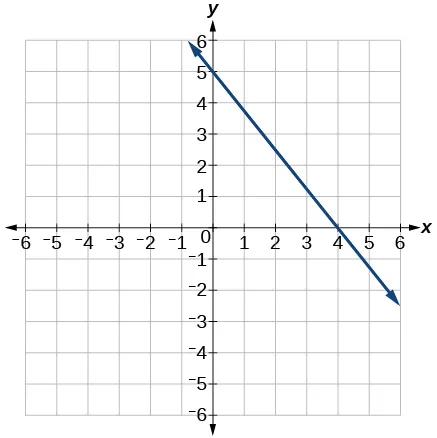57.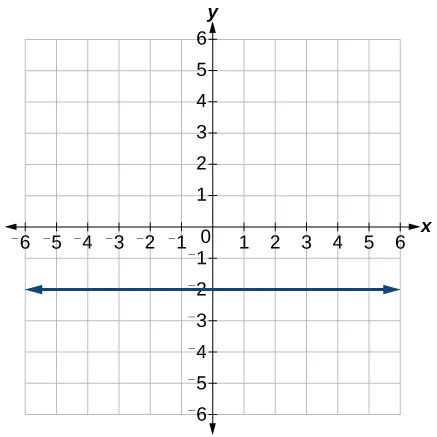For the following exercises, write an equation for the line graphed.

58.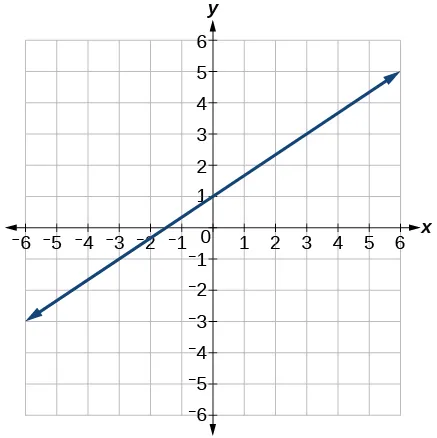59.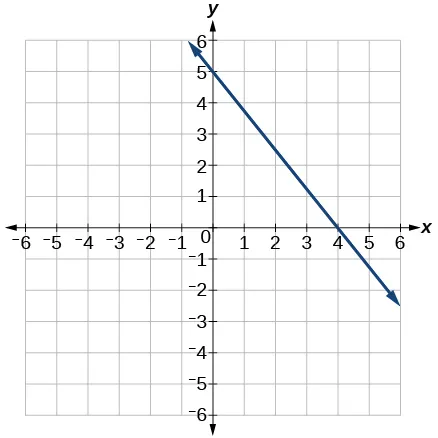60.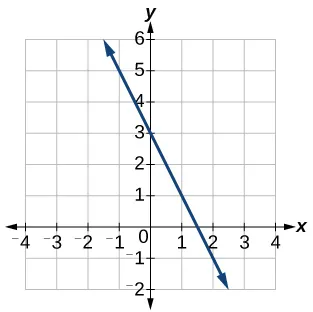61.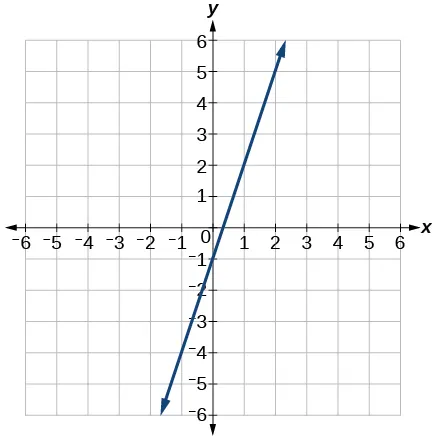62.63.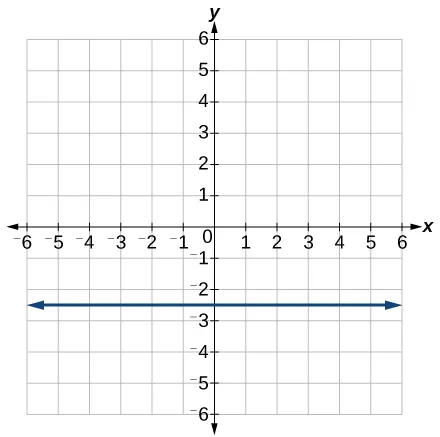For the following exercises, match the given linear equation with its graph in Figure 33.

Figure 33
64.

$f( x )=−x−1 f( x )=−x−1$

65.

$f( x )=−3x−1 f( x )=−3x−1$

66.

$f( x )=− 1 2 x−1 f( x )=− 1 2 x−1$

67.

$f( x )=2 f( x )=2$

68.

$f( x )=2+x f( x )=2+x$

69.

$f( x )=3x+2 f( x )=3x+2$

For the following exercises, sketch a line with the given features.

70.

An x-intercept of $(–4,0) (–4,0)$ and y-intercept of $(0,–2) (0,–2)$

71.

An x-intercept $(–2,0) (–2,0)$ and y-intercept of $(0,4) (0,4)$

72.

A y-intercept of $(0,7) (0,7)$ and slope $− 3 2 − 3 2$

73.

A y-intercept of $(0,3) (0,3)$ and slope $2 5 2 5$

74.

Passing through the points $(–6,–2) (–6,–2)$ and $(6,–6) (6,–6)$

75.

Passing through the points $(–3,–4) (–3,–4)$ and $(3,0) (3,0)$

For the following exercises, sketch the graph of each equation.

76.

$f( x )=−2x−1 f( x )=−2x−1$

77.

$f( x )=−3x+2 f( x )=−3x+2$

78.

$f( x )= 1 3 x+2 f( x )= 1 3 x+2$

79.

$f( x )= 2 3 x−3 f( x )= 2 3 x−3$

80.

$f( t )=3+2t f( t )=3+2t$

81.

$p( t )=−2+3t p( t )=−2+3t$

82.

$x=3 x=3$

83.

$x=−2 x=−2$

84.

$r( x )=4 r( x )=4$

For the following exercises, write the equation of the line shown in the graph.

85.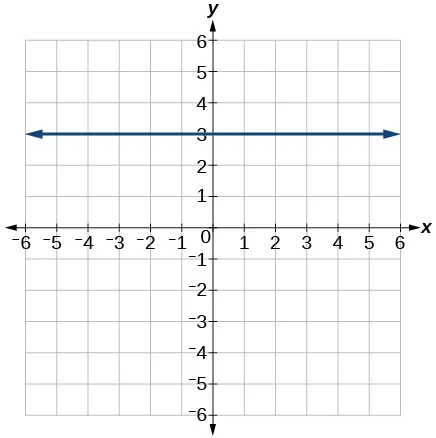86.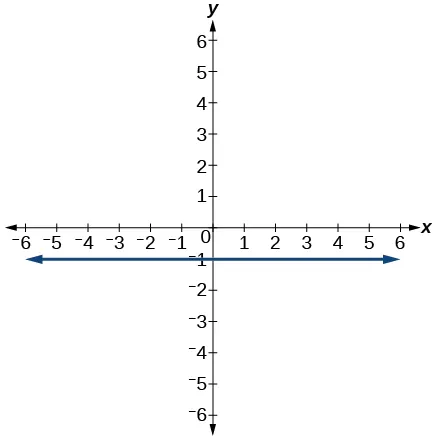87.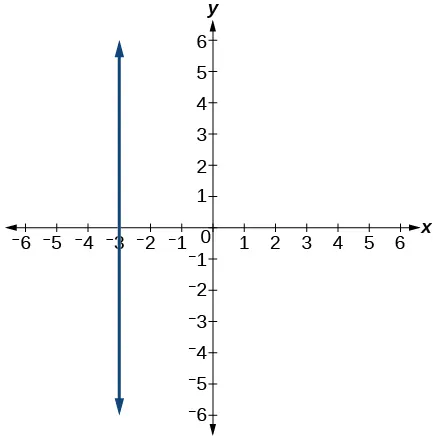88.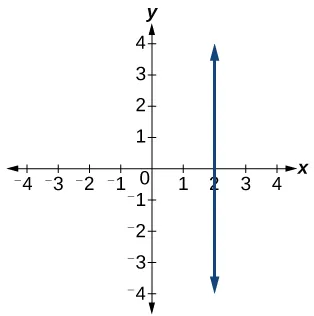#### Numeric

For the following exercises, which of the tables could represent a linear function? For each that could be linear, find a linear equation that models the data.

89.
 $x x$ 0 5 10 15 $g( x ) g( x )$ 5 –10 –25 –40
90.
 $xx$ 0 5 10 15 $h( x ) h( x )$ 5 30 105 230
91.
 $x x$ 0 5 10 15 $f( x ) f( x )$ –5 20 45 70
92.
 $x x$ 5 10 20 25 $k( x ) k( x )$ 13 28 58 73
93.
 $x x$ 0 2 4 6 $g( x ) g( x )$ 6 –19 –44 –69
94.
 $x x$ 2 4 8 10 $h( x ) h( x )$ 13 23 43 53
95.
 $x x$ 2 4 6 8 $f( x ) f( x )$ –4 16 36 56
96.
 $x x$ 0 2 6 8 $k( x ) k( x )$ 6 31 106 231

#### Technology

For the following exercises, use a calculator or graphing technology to complete the task.

97.

If $f f$ is a linear function, $f(0.1)=11.5f(0.1)=11.5$ , and $f(0.4)=–5.9 f(0.4)=–5.9$ , find an equation for the function.

98.

Graph the function $f f$ on a domain of $[–10,10]:f(x)=0.02x−0.01. [–10,10]:f(x)=0.02x−0.01.$ Enter the function in a graphing utility. For the viewing window, set the minimum value of $x x$ to be $−10 −10$ and the maximum value of $x x$ to be $10 10$ .

99.

Graph the function $f f$ on a domain of $[–10,10]:fx)=2,500x+4,000 [–10,10]:fx)=2,500x+4,000$

100.

Table 3 shows the input, $w, w,$ and output, $k, k,$ for a linear function $k. k.$

1. Fill in the missing values of the table.
2. Write the linear function

$k, k,$ round to 3 decimal places.

 w –10 5.5 67.5 b k 30 –26 a –44
Table 3
101.

Table 4 shows the input, $p, p,$ and output, $q, q,$ for a linear function $q. q.$

1. Fill in the missing values of the table.
2. Write the linear function

$k. k.$

 p 0.5 0.8 12 b q 400 700 a 1,000,000
Table 4
102.

Graph the linear function $f f$ on a domain of $[ −10,10 ] [ −10,10 ]$ for the function whose slope is $1 8 1 8$ and y-intercept is $31 16 . 31 16 .$ Label the points for the input values of $−10 −10$ and $10. 10.$

103.

Graph the linear function $f f$ on a domain of $[ −0.1,0.1 ] [ −0.1,0.1 ]$ for the function whose slope is 75 and y-intercept is $−22.5. −22.5.$ Label the points for the input values of $−0.1 −0.1$ and $0.1. 0.1.$

104.

Graph the linear function $f f$ where $f( x )=ax+b f( x )=ax+b$ on the same set of axes on a domain of $[ −4,4 ] [ −4,4 ]$ for the following values of $a a$ and $b. b.$

1. $a=2;b=3 a=2;b=3$
2. $a=2;b=4 a=2;b=4$
3. $a=2;b=–4 a=2;b=–4$
4. $a=2;b=–5 a=2;b=–5$

#### Extensions

105.

Find the value of $x x$ if a linear function goes through the following points and has the following slope: $(x,2),(−4,6),m=3 (x,2),(−4,6),m=3$

106.

Find the value of y if a linear function goes through the following points and has the following slope: $(10,y),(25,100),m=−5 (10,y),(25,100),m=−5$

107.

Find the equation of the line that passes through the following points:

$( a,b ) ( a,b )$ and $( a,b+1 ) ( a,b+1 )$

108.

Find the equation of the line that passes through the following points:

$(2a,b) (2a,b)$ and $(a,b+1) (a,b+1)$

109.

Find the equation of the line that passes through the following points:

$(a,0) (a,0)$ and $(c,d) (c,d)$

110.

Find the equation of the line parallel to the line $g( x )=−0.01x+2.01 g( x )=−0.01x+2.01$ through the point $(1,2). (1,2).$

111.

Find the equation of the line perpendicular to the line $g( x )=−0.01x+2.01 g( x )=−0.01x+2.01$ through the point $(1,2). (1,2).$

For the following exercises, use the functions

112.

Find the point of intersection of the lines $f f$ and $g. g.$

113.

Where is $f( x ) f( x )$ greater than $g( x )? g( x )?$ Where is $g( x ) g( x )$ greater than $f( x )? f( x )?$

#### Real-World Applications

114.

At noon, a barista notices that they have $20 in their tip jar. If the barista makes an average of$0.50 from each customer, how much will they have in the tip jar if they serve $nn$ more customers during the shift?

115.

A gym membership with two personal training sessions costs $125, while gym membership with five personal training sessions costs$260. What is cost per session?

116.

A clothing business finds there is a linear relationship between the number of shirts, $n, n,$ it can sell and the price, $p, p,$ it can charge per shirt. In particular, historical data shows that 1,000 shirts can be sold at a price of $30, 30,$ while 3,000 shirts can be sold at a price of $22. Find a linear equation in the form $p(n)=mn+b p(n)=mn+b$ that gives the price $p p$ they can charge for $n n$ shirts. 117. A phone company charges for service according to the formula: $C(n)=24+0.1n, C(n)=24+0.1n,$ where $n n$ is the number of minutes talked, and $C(n) C(n)$ is the monthly charge, in dollars. Find and interpret the rate of change and initial value. 118. A farmer finds there is a linear relationship between the number of bean stalks, $n, n,$ she plants and the yield, $y, y,$ each plant produces. When she plants 30 stalks, each plant yields 30 oz of beans. When she plants 34 stalks, each plant produces 28 oz of beans. Find a linear relationships in the form $y=mn+b y=mn+b$ that gives the yield when $n n$ stalks are planted. 119. A city’s population in the year 1960 was 287,500. In 1989 the population was 275,900. Compute the rate of growth of the population and make a statement about the population rate of change in people per year. 120. A town’s population has been growing linearly. In 2003, the population was 45,000, and the population has been growing by 1,700 people each year. Write an equation, $P(t), P(t),$ for the population $t t$ years after 2003. 121. Suppose that average annual income (in dollars) for the years 1990 through 1999 is given by the linear function: $I(x)=1054x+23,286 I(x)=1054x+23,286$, where $x x$ is the number of years after 1990. Which of the following interprets the slope in the context of the problem? 1. As of 1990, average annual income was$23,286.
2. In the ten-year period from 1990–1999, average annual income increased by a total of $1,054. 3. Each year in the decade of the 1990s, average annual income increased by$1,054.
4. Average annual income rose to a level of \$23,286 by the end of 1999.
122.

When temperature is 0 degrees Celsius, the Fahrenheit temperature is 32. When the Celsius temperature is 100, the corresponding Fahrenheit temperature is 212. Express the Fahrenheit temperature as a linear function of $C, C,$ the Celsius temperature, $F( C ). F( C ).$

1. Find the rate of change of Fahrenheit temperature for each unit change temperature of Celsius.
2. Find and interpret $F(28). F(28).$
3. Find and interpret $F(–40). F(–40).$

### Footnotes

• 2http://www.chinahighlights.com/shanghai/transportation/maglev-train.htm
• 3http://www.cbsnews.com/8301-501465_162-57400228-501465/teens-are-sending-60-texts-a-day-study-says/
Order a print copy

As an Amazon Associate we earn from qualifying purchases.